## Recommended Posts

It has been a long time since I've posted one of my challenges. The reason is not because a lack of time. Instead, I am running out of problems that can be considered interesting or at least require some skill in solving mathematical puzzles. Sure, I could try to find something on the internet, but I don't want you to be able to simply look up the answer, and I want the problems to really make you think about possible solutions so that you discover something new for yourself, which is way more rewarding than having someone teach you math.

For those of you that have never seen them, here are the links to my previous challenges (the first challenge is my favorite, but I also really like the second and fourth challenge too):

Daedalus' First Challenge
Daedalus' Second Challenge
Daedalus' Third Challenge
Daedalus' Fourth Challenge
Daedalus' Fifth Challenge

My sixth challenge is based on the Collatz conjecture. Wikipedia provides a decent explanation of the problem:

Take any natural number n. If n is even, divide it by 2 to get n / 2. If n is odd, multiply it by 3 and add 1 to obtain 3n + 1. Repeat the process ... indefinitely. The conjecture is that no matter what number you start with, you shall always eventually reach 1.

The problem is also defined by Wolfram as:

Let $a_0$ be an integer. Then one form of Collatz problem asks if iterating

$a_n=\begin{cases}\frac{1}{2}a_{n-1},&\text{for }a_{n-1}\text{ even}\\3\,a_{n-1}+1,&\text{for }a_{n-1}\text{ odd}\end{cases}$

always returns to 1 for positive $a_0$

Although I haven't proved the conjecture, I have discovered that I can predict the odd numbers that reduce to one given the count of odd numbers contained within their Hailstone sequence. For instance, the number 3 has three odd numbers in its Hailstone sequence: {3, 10, 5, 16, 8, 4, 2, 1}. However, I cannot easily predict the count of odd numbers contained within the Hailstone sequence of a given odd number.

The challenge is to provide the equation(s) for the odd numbers that reduce to one given a count of two odd numbers in their Hailstone sequence as well as a count of three odd numbers in their Hailstone sequence. As usual, I will give ample time to find the solution and give reputation to the one who solves it first.

Edited by Daedalus

##### Share on other sites

Anyone going to try to figure this one out?

I got 2 in about 5-10 minutes, but I'm doing something wrong with 3, because I'm pretty sure I know what should work, but it doesn't seem to. Once I sit down with a pencil and paper instead of staring at the screen trying to work it out mentally, it'll probably go better.

##### Share on other sites

I got 2 in about 5-10 minutes, but I'm doing something wrong with 3, because I'm pretty sure I know what should work, but it doesn't seem to. Once I sit down with a pencil and paper instead of staring at the screen trying to work it out mentally, it'll probably go better.

Yeah, the equation for two odd numbers is easy to find once you realize what happens to the even numbers. Three odd numbers in the Hailstone sequence is a bit more challenging ; )

##### Share on other sites

## I lack skills to do any kind of formal analysis but it is an iteresting question it seems obvious that every list should end, but I couldn't prove it!
##If I didn't make a mistake, this should calculate the lists for every number 1-MAXARG
##save the length of the list of iterated values in a list of lengths
##print out the length of the longest list encountered at powers of ten (longest list of iterates from interval 1-10,1-100,1-1000 etc.)
##With a little modification, the code will also print the lists of iterated values (print iterVals)
##

MAXARG = 10000000

## itlist: return a list of iterated values for the argument
def itlist(val):
tlist = []
n=val
while n > 1:
tlist.append(n)
if n & 1:
n = (3 * n) + 1
else:
n = n/2
tlist.append(n)
return tlist

##========================================
## Find the length of the iterated values lists for 1 through MAXARG

countList = [] ## list to hold iterated values from seed
listLen = 0 ## number of iterated values on list
seed = 1 ## seed value to generate list
pow = 10 ## threshold value to check length
max = 0
while seed <= MAXARG:
iterVals = itlist(seed)
listLen = len(iterVals)
countList.append(listLen)
if listLen > max: max = listLen
if seed == pow:
print pow,max
pow = pow * 10

seed = seed + 1

output:

10 20
100 119
1000 179
10000 262
100000 351
1000000 525
10000000 686

##### Share on other sites

Moth I don't think your program is producing the correct results. Check the output with a known Hailstone sequence. I would check it for you, but I'm at work. However, this challenge requires both algebra and analytic methods to find the solutions.

##### Share on other sites

The comments in the code are not correct(apologies). I started this and then houseguests showed up...
This is the first 10 lists. they seem ok

#!/bin/usr/python
MAXARG = 10
## itlist: return a list of iterated values for the argument
def itlist(val):
tlist = []
n=val
while n > 1:
tlist.append(n)
if n & 1:
n = (3 * n) + 1
else:
n = n/2
tlist.append(n)
return tlist

##========================================
## Find the length of the iterated values lists for 1 through MAXARG

countList = [] ## list to hold list length for iterated values from seed
listLen = 0 ## number of iterated values on list
seed = 1 ## seed value to generate list
pow = 10 ## threshold value to check length
max = 0
while seed <= MAXARG:
iterVals = itlist(seed)
listLen = len(iterVals)
print iterVals
countList.append(listLen)
if listLen > max: max = listLen
if seed == pow:
## print pow,max
pow = pow * 10

seed = seed + 1

OUTPUT:

[2, 1]
[3, 10, 5, 16, 8, 4, 2, 1]
[4, 2, 1]
[5, 16, 8, 4, 2, 1]
[6, 3, 10, 5, 16, 8, 4, 2, 1]
[7, 22, 11, 34, 17, 52, 26, 13, 40, 20, 10, 5, 16, 8, 4, 2, 1]
[8, 4, 2, 1]
[9, 28, 14, 7, 22, 11, 34, 17, 52, 26, 13, 40, 20, 10, 5, 16, 8, 4, 2, 1]
[10, 5, 16, 8, 4, 2, 1]

##### Share on other sites

Moth, those results look a lot better than the outputs you posted previously. As for solving the challenge, try to find the equation for the odd numbers that only have two odd numbers in their Hailstone sequence. Once you have done that, then the next part becomes obvious but still challenging.

Edited by Daedalus

##### Share on other sites

Thanks Daedalus, I'm glad I may be not too far off the track.
The output from the first post was showing how long the longest hailstone sequence is for the starting values between 1 and 10, 1 and 100, etc.
The output shows (IF i didn't pooch it) that the longest hailstone sequence found for initial values less than 10000000 is 686 iterations long (for the number 8400511 not shown)

##### Share on other sites

I got...

2 odd numbers:

$(2^{(2i+2)} - 1)/3$

for i in {1, 2, 3, ...}

3 odd:

$(((2^{(6i-2)}-1)/3 * 2^{(2j+1)})-1)/3$

for i and j in {1, 2, 3, ...}

##### Share on other sites

I got...

2 odd numbers:

$(2^{(2i+2)} - 1)/3$

for i in {1, 2, 3, ...}

3 odd:

$(((2^{(6i-2)}-1)/3 * 2^{(2j+1)})-1)/3$

for i and j in {1, 2, 3, ...}

Almost there md65536. Although your equations are correct, you are missing an equation for 3 odd numbers in the Hailstone sequence. Thus, the solution for 3 odd numbers requires 2 functions.

Edited by Daedalus

##### Share on other sites

Almost there md65536. Although your equations are correct, you are missing an equation for 3 odd numbers in the Hailstone sequence. Thus, the solution for 3 odd numbers requires 2 functions.

Is there an example number that I missed?

Before you answer!, I noticed I made an error in the equation:

3 odd:

$(((2^{(6i-2)}-1)/3 * 2^{(2j-1)})-1)/3$

for i and j in {1, 2, 3, ...}

The previous answer I gave was using values for j starting at 0.

##### Share on other sites

Is there an example number that I missed?Before you answer!, I noticed I made an error in the equation:

3 odd:$(((2^{(6i-2)}-1)/3 * 2^{(2j-1)})-1)/3$for i and j in {1, 2, 3, ...}The previous answer I gave was using values for j starting at 0.

I checked your equations earlier and they produced the correct results. I'm not concerned with indices being offset as long as the equations produce the correct sequences. As for the missing equation for 3 odd numbers, the pattern is a little more complex. I'm on my phone right now, but when I get home tonight I will post a sequence that the equation you posted for 3 odd numbers doesn't predict. In other words, not only do the dimensions increase as there are more odd numbers in the Hailstone sequence, but also the number of equations too.

As for an example number, it's either 13 or 113. Both have 3 odd numbers, but since I'm not home I can't check which one belongs to the missing equation.

Edited by Daedalus

##### Share on other sites

As for an example number, it's either 13 or 113. Both have 3 odd numbers, but since I'm not home I can't check which one belongs to the missing equation.

Yep, it's 113.

3 odd:

$(((2^{(6i-2)}-1)/3 * 2^{(2j-1)})-1)/3$

and

$(((2^{(6i+2)}-1)/3 * 2^{(2j)})-1)/3$

for i and j in {1, 2, 3, ...}

I get the sense that this could be massaged into one equation?

I couldn't remember how I arrived at the answer I came up with last night, haha. But now I see it was partly guess-and-check, and partly figuring out why every third value of i works with 2^(i+2). Still not sure how this works; to figure it out appears to require 1 more than the maximum number of things I can keep in my head.

##### Share on other sites

Md65536, I'll check your equations when I get home tonight. At first glance, they seem to be correct. If so, then i'll post the usual breakdown and explanation of the solution. There are two main patterns that are expressed for the indices with some interesting consequences as a result.

Although it might be possible to massage these equations into one, it will be extremely difficult because the number of equations increase nonlinearly as the number of odd numbers in the Hailstone sequence increases.

Edited by Daedalus

##### Share on other sites

Good job md65536, you provided the correct solutions and won the challenge earning you rep and 5 stars from me : )

Edited by Daedalus

##### Share on other sites

Good job md65536, you provided the correct solutions and won the challenge earning you rep and 5 stars from me : )##### Share on other sites

When I first started working on trying to prove the Collatz conjecture I noticed that every single even number will reduce to an odd number, and that even numbers of the form $2^x$ will reduce to one without incurring another odd number in the Hailstone sequence. So the first thing I did was to set

$3\,m+1=2^n$

Solving for $m$ we get

$m=\frac{2^n-1}{3}$

However, not all values of $n$ produce odd numbers. Instead, every other number is odd with the rest being rational numbers as shown in the following image: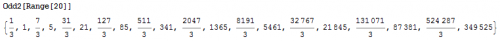As we can see, every other number produces an odd number. Thus, we have the first pattern in the form of skipping every other number, and arrive at the equation for all of the odd numbers that reduces to one which have two odd numbers in their Hailstone sequence:

$m=\frac{2^{2\,n}-1}{3}$

It became obvious that if the conjecture was true, then all other odd numbers must eventually reduce to one of the values of $m$, which would then reduce to 1. I realized that I could repeat the process, but that I would have to add a new dimension for each $2^x$ that was nested. Thus, I arrived at the following generalization: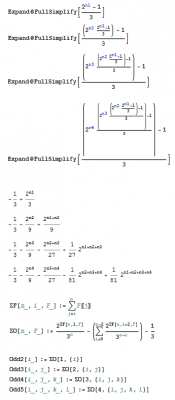Every single odd number that reduces to one is determined by the above generalization. However, the challenge is now finding the equations for the indices of each dimension. By analyzing the equation for 3 odd numbers, I was able to find the second and final pattern (the one that generates the $6\,x + \text{offset}$ for the remaining variables):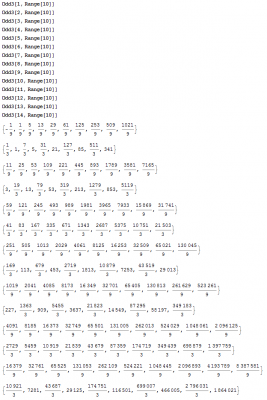You can see that rows 2, 4, 8, 10, 14, 16, etc... all have odd numbers that reduce to one of the numbers in the first equation. However, it's easier to write an equation for 2, 8, 14, etc... and one for 4, 10, 16, etc... This produces the following equations for the indices:

$6\,x-4$

$6\,x-2$

This is why there are two equations for 3 odd numbers: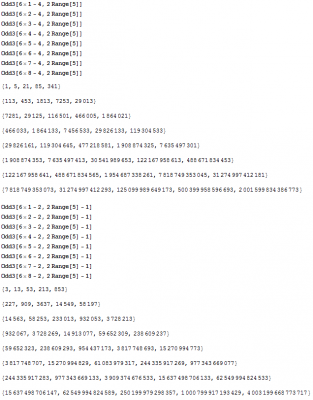The above image shows us why we either have to skip every other number by $2\,x$ or $2\,x-1$, which further complicates the problem. However, the rest of the indices are of the form $6\,x-\text{offset}$. I've checked this for 4 and 5 odd numbers in the sequence and it is holding true.

You might have noticed that we got back the equation for two odd numbers in the Hailstone sequence in our solutions for three odd numbers in the Hailstone sequence. Although this may seem odd, you have to remember that Hailstone sequences are cyclic when they reach one. This is because if you plug 1 back into the formula, you will get back 1 again.

Now for the most interesting thing of all. You would think that the odd numbers that have three odd numbers in their Hailstone sequence would reduce to one of the odd numbers that have two odd numbers in their Hailstone sequence and that you would get back the entire set of numbers. However, this isn't the case. It turns out that there are odd numbers that have two odd numbers in their Hailstone sequence that do not branch to an odd number that has three odd numbers in its sequence. I discovered this fact when I applied the Collatz formula to the odd numbers that have three odd numbers in its sequence to produce:As you can see, these odd numbers do not reduce to every odd number that has two odd numbers in their Hailstone sequence. In other words, the numbers 21, 1365, 87381, etc... do not branch to an odd number that has 3 odd numbers in its sequence. In fact, any odd number that has two odd numbers in its Hailstone sequence and is a multiple of 3 will not branch to an odd number that has 3 odd numbers in its Hailstone sequence. I propose that such numbers terminate that branch and will not produce another odd number in the sequence.

I have attached a zipped Mathematica 7 file containing the work I've done thus far to this post for anyone who wishes to work with me or explore the problem for yourself. All I ask is that if you do manage to prove the conjecture using my work, that you give credit where it is due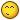Collatz.zip

Edited by Daedalus

##### Share on other sites

All I ask is that if you do manage to prove the conjecture using my work, that you give credit where it is dueOh boy... I think it's safe!

I've thought of this conjecture a few times over the years, never with this much mathematical analysis. When I first heard of it, it was presented as an algorithm to demonstrate the idea of processes that you couldn't say whether or not would terminate. I think a prof said something like, if you can prove that it would (or not) on every input, you'd become rich. So it piqued my interest!

I think the prof also said that to prove it would likely require a whole new type of math, which is interesting because new types of math are sometimes invented to deal with physical problems (eg. calculus). As we understand the world in greater details and scales, we need new maths to describe it.

The way that I imagine this conjecture being proved is through some kind of quantum computer algorithm, using superposition... If you could define mathematical operators that represent the results of both operations (x/2 or 3x+1) at the same time, and then are able to analyze the results of infinite iterations of the operator, then you might be able to prove it?

##### Share on other sites

The answer to the conjecture is beyond me, so if one of us solves it that would probably be you or md.
I was looking at how long the even-odd-even-odd pattern needed for fastest growth of n could be sustained, and if some property of multiples of two, when constrained by the 3n+1 rule, might interfere.
+1 for the question and 1 for your exposition of your analysis.

##### Share on other sites

Oh boy... I think it's safe!

I've thought of this conjecture a few times over the years, never with this much mathematical analysis. When I first heard of it, it was presented as an algorithm to demonstrate the idea of processes that you couldn't say whether or not would terminate. I think a prof said something like, if you can prove that it would (or not) on every input, you'd become rich. So it piqued my interest!

I think the prof also said that to prove it would likely require a whole new type of math, which is interesting because new types of math are sometimes invented to deal with physical problems (eg. calculus). As we understand the world in greater details and scales, we need new maths to describe it.

The way that I imagine this conjecture being proved is through some kind of quantum computer algorithm, using superposition... If you could define mathematical operators that represent the results of both operations (x/2 or 3x+1) at the same time, and then are able to analyze the results of infinite iterations of the operator, then you might be able to prove it?

I also heard the same things from my prof, which is why I applied the depth of analysis that I have done and continue to do. Although I was unable to prove the conjecture, I think the work I've done so far is absolutely awesome. Of course, I love everything mathematical and this conjecture yielded some unexpected results, which, as you know, is what us mathematicians live for and strive to achieve. To think that the dimensionality of the equations that predict these odd numbers approach infinity is a mind boggling result. Not to mention that the number of equations also increase. Furthermore, the equations that predict these odd numbers include the equations for the previous odd numbers. This means that if you could somehow take this process to infinity, not only would you have equations with infinite dimensions, but also that there would be an infinite number of such equations. Truly an amazing result!

The answer to the conjecture is beyond me, so if one of us solves it that would probably be you or md.

I was looking at how long the even-odd-even-odd pattern needed for fastest growth of n could be sustained, and if some property of multiples of two, when constrained by the 3n+1 rule, might interfere.

+1 for the question and 1 for your exposition of your analysis.

Moth, I commend your efforts and give you props. Thanks for the rep. It truly feels like a pat on the back. Although this problem was beyond your current level of mathematical skill, I do hope that you were able to take from this challenge and increase your level of understanding on how we attack these type of problems. Just remember that everything you have ever learned in math is just another puzzle piece in the big picture. As you tackle and solve mathematical problems, you gain new puzzle pieces that can help you solve the next problem. Even if you fail to solve a problem, you can still gain valuable insights into mathematics by just making the attempt.

Edited by Daedalus

##### Share on other sites

The answer to the conjecture is beyond me, so if one of us solves it that would probably be you or md.

I was looking at how long the even-odd-even-odd pattern needed for fastest growth of n could be sustained, and if some property of multiples of two, when constrained by the 3n+1 rule, might interfere.

+1 for the question and 1 for your exposition of your analysis.

Oh jeez... it certainly won't be me! ...not unless I invent a new form of math that I understand better than normal maths, or I get zapped by a Cytherian probe and then Geordi gets all jealous when I'm super smart.

I'll explain a bit how I solved it to show it didn't require difficult math.

First of all I made a list of "1-odd" (ignoring whether odd or even), which are {1, 2, 4, 8, 16, ...}, and realized that any in 2-odd must be one of those numbers x, less one, divided by 3. That is, keep any where x-1 is divisible by 3.

Here's where I started CHEATING... I put it all in a Libreoffice spreadsheet and made a grid of numbers to look for patterns. Then the pattern where alternating numbers are divisible is readily apparent. However you can easily prove that the pattern will continue, because if x-1 = 3b for some natural b, then 4x-1 = 4(3b+1)-1 = 12b+3 is divisible by 3, but 2x-1 = 6b+1 is clearly not.

Then repeat the reasoning... 3-odd must be one of 2-odd, multiplied by some 2^j, minus 1 divided by 3, and then only if it's divisible by 3. So I made a 2d grid with increasing values of i and j and filled it with a naive formula that didn't care if it was divisible or not, and looked for patterns. The non-divisible ones have a bunch of decimal places. Here the "every third row is integral" pattern shows up, and you can again easily prove that that pattern continues.

I think I accidentally copied the "skip every second value" and put in the wrong formula, which is why I missed the second formula (but I think I lucked out because the grid with all of the values in it looks too hard to figure out. I would have been stuck, looking for a single equation). After Daedalus corrected me I just wrote out the 113 sequence and fiddled with the formula until it produced it. :S

Once the simple "every third value of i works" pattern is established, you just have to multiply i by 3 with the correct offset.

Being able to find the pattern with brute force is one thing, being able to show that it works for all values is pretty good I guess, but understanding why it works is another thing! After Daedalus' explanation I can almost uh... imagine understanding it.

Edited by md65536

##### Share on other sites

Oh jeez... it certainly won't be me! ...not unless I invent a new form of math that I understand better than normal maths, or I get zapped by a Cytherian probe and then Geordi gets all jealous when I'm super smart.

Funny that you mentioned the Cytherian probe because I just watched that episode last night. It's one of my favorites. However, if I was Lieutenant Barclay, I would've had the computer record every single thought that came to me.

I'll explain a bit how I solved it to show it didn't require difficult math.

First of all I made a list of "1-odd" (ignoring whether odd or even), which are {1, 2, 4, 8, 16, ...}, and realized that any in 2-odd must be one of those numbers x, less one, divided by 3. That is, keep any where x-1 is divisible by 3.

Here's where I started CHEATING... I put it all in a Libreoffice spreadsheet and made a grid of numbers to look for patterns. Then the pattern where alternating numbers are divisible is readily apparent. However you can easily prove that the pattern will continue, because if x-1 = 3b for some natural b, then 4x-1 = 4(3b+1)-1 = 12b+3 is divisible by 3, but 2x-1 = 6b+1 is clearly not.

Then repeat the reasoning... 3-odd must be one of 2-odd, multiplied by some 2^j, minus 1 divided by 3, and then only if it's divisible by 3. So I made a 2d grid with increasing values of i and j and filled it with a naive formula that didn't care if it was divisible or not, and looked for patterns. The non-divisible ones have a bunch of decimal places. Here the "every third row is integral" pattern shows up, and you can again easily prove that that pattern continues.

I think I accidentally copied the "skip every second value" and put in the wrong formula, which is why I missed the second formula (but I think I lucked out because the grid with all of the values in it looks too hard to figure out. I would have been stuck, looking for a single equation). After Daedalus corrected me I just wrote out the 113 sequence and fiddled with the formula until it produced it. :S

Once the simple "every third value of i works" pattern is established, you just have to multiply i by 3 with the correct offset.

Being able to find the pattern with brute force is one thing, being able to show that it works for all values is pretty good I guess, but understanding why it works is another thing! After Daedalus' explanation I can almost uh... imagine understanding it.

I'm glad that you mentioned solving the pattern using brute force as well as provide your method for solving the challenge. Although I found a generalized algorithm that encompasses the problem set, I still had to resort to using brute force to find the patterns myself. It's important for an upcomming mathematician to understand that not all problems can be solved by manipulating equations alone. One must be able to search for patterns in a sea of numbers and variables, and to recognize the equations that predict how such patterns behave. Again, good job md65536!

Edited by Daedalus

##### Share on other sites

To think that the dimensionality of the equations that predict these odd numbers approach infinity is a mind boggling result. Not to mention that the number of equations also increase. Furthermore, the equations that predict these odd numbers include the equations for the previous odd numbers. This means that if you could somehow take this process to infinity, not only would you have equations with infinite dimensions, but also that there would be an infinite number of such equations. Truly an amazing result!

Hrm, I guess I can see how that could be useful. The intersection of any of 1-odd, 2-odd, 3-odd, etc is empty.

But does that get you anywhere toward proving that all odd numbers are covered by those sets?

Is it possible to determine the cardinality of all infinity of those sets up to a given limit N, and show that it's equal to N/2?

Is there a formula for the cardinality of the sets?

They have infinite dimensions but can't you map the multiple dimensions to 1 using the "diagonal processing" method (http://www.proofwiki.org/wiki/Cartesian_Product_of_Countable_Sets_is_Countable)?

##### Share on other sites

Hrm, I guess I can see how that could be useful. The intersection of any of 1-odd, 2-odd, 3-odd, etc is empty.

But does that get you anywhere toward proving that all odd numbers are covered by those sets?

Is it possible to determine the cardinality of all infinity of those sets up to a given limit N, and show that it's equal to N/2?

Is there a formula for the cardinality of the sets?

They have infinite dimensions but can't you map the multiple dimensions to 1 using the "diagonal processing" method (http://www.proofwiki.org/wiki/Cartesian_Product_of_Countable_Sets_is_Countable)?

Before I can answer any of these questions, I will have to derive the formula that will predict the equations. For instance, I still do not know the formula that predicts the count of the equations as the dimensionality increases, and I'm not sure if such approach will allow me to prove that all odd numbers are covered by these sets. As you are well aware, managing all of these equations becomes extremely difficult and it is easy for one to miss an equation within the set. However, since I do have a generalized formula for deriving such sets, the next step is to develop software that will iterate through each one and then develop a formula based on the results. To accomplish this, I actually use MathLink to invoke Mathematica within a C# application, which for me is easier than trying to program Mathematica itself. The two main patterns are skipping every other number $2\,x_0 - \text{offset}_0$ and $6\,x_i - \text{offset}_i$. Once I am able to predict the offset for each index as the dimensionality grows, then I might be able to answer some of these questions.

Edited by Daedalus

## Create an account

Register a new account